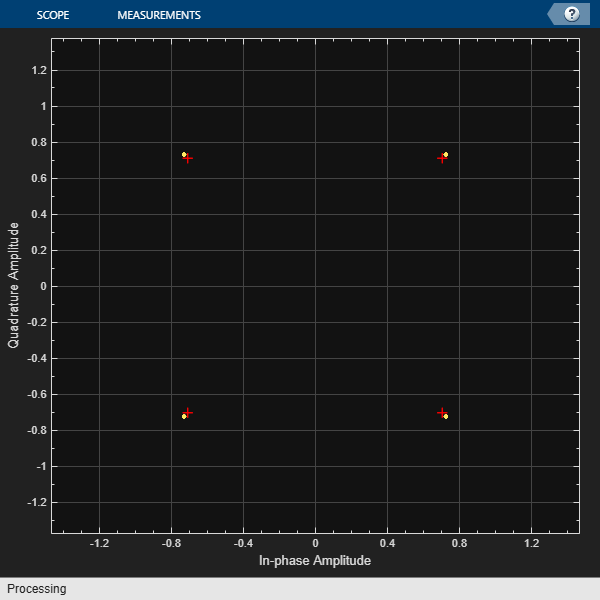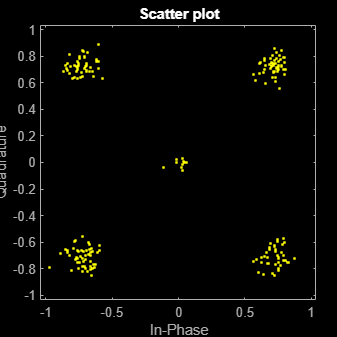## Scatter Plots and Constellation Diagrams

Scatter plots and constellation diagrams display the constellation of digitally modulated signals in the IQ-plane. Specifically, the IQ-plane displays the in-phase and quadrature components of the modulated signal on the real and imaginary axis of an xy-plot.

To produce a scatter plot from a signal, use the `scatterplot` function, the `comm.ConstellationDiagram` System object™, or the Constellation Diagram block. A scatter plot or constellation diagram can be useful when comparing system performance and the effects of channel and RF impairments.

### View Signals Using Constellation Diagrams

This example shows how to use constellation diagrams to view QPSK transmitted and received signals which are pulse shaped with a raised cosine filter.

Create a QPSK modulator.

```qpsk = comm.QPSKModulator; ```

Create a raised cosine transmit filter with samples per symbol, `sps`, equal to 16.

```sps = 16; txfilter = comm.RaisedCosineTransmitFilter('Shape','Normal', ... 'RolloffFactor',0.22, ... 'FilterSpanInSymbols',20, ... 'OutputSamplesPerSymbol',sps); ```

Generate data symbols, apply QPSK modulation, and pass the modulated data through the raised cosine transmit filter.

```data = randi([0 3],200,1); modData = qpsk(data); txSig = txfilter(modData); ```

You can display the constellation diagram of the transmitted signal using `scatterplot`. Since the signal is oversampled at the filter output, you need to decimate by the number of samples per symbol so that the scatter plot does not show the transition path between constellation points. If the signal had a timing offset, you could provide that as an input parameter to display the signal constellation with the timing offset corrected.

```scatterplot(txSig,sps) ```Alternately, you can use `comm.ConstellationDiagram`, specifying the number of samples per symbol, and if needed the timing offset. Also, using `comm.ConstellationDiagram` the reference constellation can be shown.

Create a constellation diagram and set the `SamplesPerSymbol` property to the oversampling factor of the signal. Specify the constellation diagram so that it only displays the last 100 samples. This hides the zero values output by the RRC filter for the first `FilterSpanInSymbols` samples.

```constDiagram = comm.ConstellationDiagram('SamplesPerSymbol',sps, ... 'SymbolsToDisplaySource','Property','SymbolsToDisplay',100); ```

Display the constellation diagram of the transmitted signal.

```constDiagram(txSig) ```To match the signal to its reference constellation, normalize the filter by setting its gain to the square root of the `OutputSamplesPerSymbol` property. This was previously specified as `sps`. The filter gain is nontunable so the object must be released prior to changing this value.

```release(txfilter) txfilter.Gain = sqrt(sps); ```

Pass the modulated signal through the normalized filter.

```txSig = txfilter(modData); ```

Display the constellation diagram of the normalized signal. The data points and reference constellation nearly overlap.

```constDiagram(txSig) ```To view the transmitted signal more clearly, hide the reference constellation by setting the `ShowReferenceConstellation` property to `false`.

```constDiagram.ShowReferenceConstellation = false; ```

Create a noisy signal by passing `txSig` through an AWGN channel.

```rxSig = awgn(txSig,20,'measured'); ```

Show the reference constellation and plot the received signal constellation.

```constDiagram.ShowReferenceConstellation = true; constDiagram(rxSig) ```You can also use `scatterplot` to view this noisy signal but there is no built in option to add the reference constellation using `scatterplot`.

```scatterplot(rxSig,sps) ```